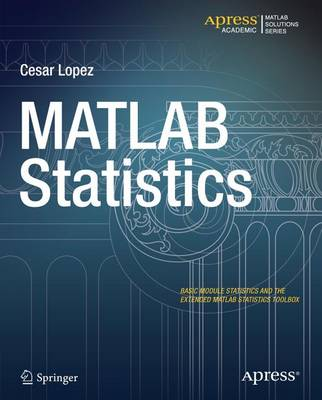Some orders may be delayed by the current Coronavirus lockdown.•# MATLAB Statistics 2016 (Paperback)

(author)
£29.50
Paperback 260 Pages / Published: 14/01/2016
• Publication abandoned

Other editions may be available

This product is currently unavailable.

MATLAB is a high-level language and environment for numerical computation, visualization, and programming. Using MATLAB, you can analyze data, develop algorithms, and create models and applications. The language, tools, and built-in math functions enable you to explore multiple approaches and reach a solution faster than with spreadsheets or traditional programming languages, such as C/C++ or Java. MATLAB Statistics introduces you to MATLAB and begins by showing you how to use the Basic Module of MATLAB statistical functions, from the elementary mathematical functions, to special mathematical functions and the functions you can use in MATLAB to perform data analysis and basic statistical analysis. You're then introduced to the MATLAB Statistics Toolbox, which extends the basic statistical functions of MATLAB, and you'll find practical hands-on examples throughout the book on how to use these extended MATLAB functions for descriptive statistics, probabilistic models, discrete and continuous random variables, confidence intervals and hypothesis contrasts, models of linear and non-linear regression, multivariate data analysis, quality control, design of experimentation and other content of industrial statistics.

Publisher: Springer-Verlag Berlin and Heidelberg GmbH & Co. KG
ISBN: 9781484202999
Number of pages: 260
Dimensions: 229 x 191 mm